# KTP（Nonlinear Crystal）

KTiOPO4 (KTP Nonlinear Crystal) has the following characteristics:

• High nonlinear coefficient (about 15 times that of KDP)
• High thermal conductivity (twice that of BDN crystal)
• A wide range of allowable temperature matching and allowable angle matching
• High threshold of resistance to gray traces and light damage
• No moisture absorption and deliquescence
• No decomposition below 900°C
• Good mechanical properties
• Easy polishing of the crystal surface and small mismatch

Its frequency-doubling efficiency for 1064 nm can reach about 80%. KTP crystal can make components for frequency doubling, frequency mixing, electro-optical modulation, optical parametric oscillation, and optical waveguide.

 Attribute Numerical Chemical Formula KTiOPO4 Crystal Structure Rhombic System, Space GroupPna21,Point Group mm2 Lattice Constant a=6.404Å, b=10.616Å, c=12.814Å, Z=8 Melting Point 1172°C Mohs Hardness 5 Density 3.01 g/cm3 Thermal Conductivity 13W/m/K Coefficient of Thermal Expansion ax=11×10-6/℃, ay=9×10-6/℃, az=0.6×10-6/℃
 Damage Threshold: [GW/cm ] >0.5 @1064 nm,TEM00, 10ns,10HZ(AR-coated) >0.3 @532 nm,TEM00, 10ns,10HZ(AR-coated) SHG Phase Matching Range 497 ~ 1800nm  (Type II) Non-vanished Nonlinear Magnetization Coefficient deff(II)≈(d24– d15)sin2φsin2θ- (d15sin2φ+ d24cos2φ)sinθ d31=6.5 pm/V d24=7.6 pm/V d32= 5 pm/V d15=6.1 pm/V d33=13.7 pm/V Thermal Coefficient of Light dnx/dT=1.1*10-5/℃ dny/dT=1.3*10-5/℃ dnz/dT=1.6*10-5/℃ Nd:YAG Type of Laser II SHG@1064nm Temperature Receiving: 24°C·cm Spectrum Receive: 0.56nm·cm Angle to Receive: 14.2mrad·cm (φ);  55.3mrad·cm(θ) Discrete Angle: 0.55°
 Transmission Range 350~4500nm Absorption Coefficient <0.1%/cm @ 1064nm；<1%/cm @ 532nm Refractive Index nx=1.7377, ny=1.7453, nz=1.8297 @1064nm nx=1.7780, ny=1.7886, nz=1.8887 @532nm Sellmeier Equation(λ in μm) nx2=3.0065+0.03901/(λ2-0.04251)-0.01327λ2 ny2=3.0333+0.04154/(λ2-0.04547)-0.01408λ2 nz2=3.3134+0.05694/(λ2-0.05658)-0.01682λ2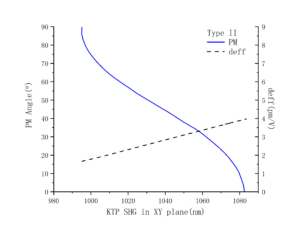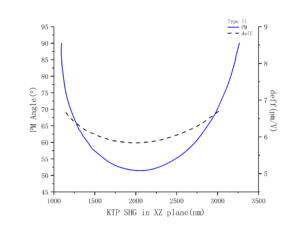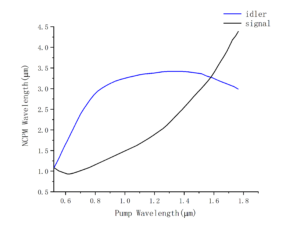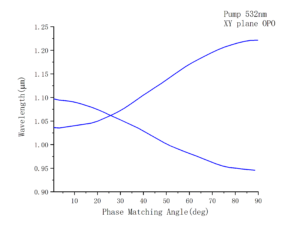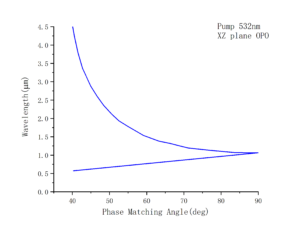## Related Posts

### would be happy to meet you and learn all about your requirements & expectations.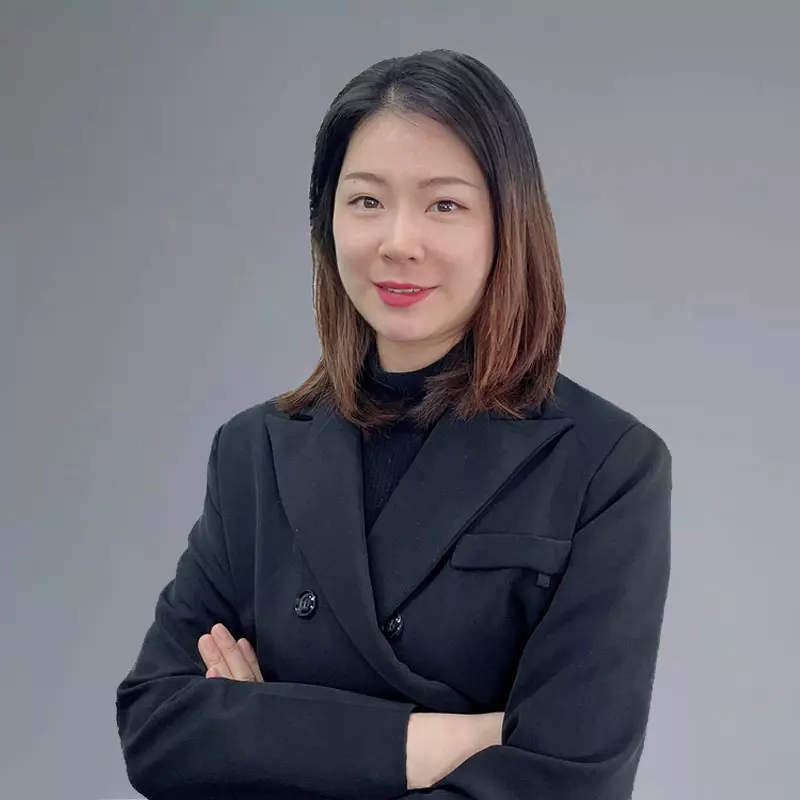Celia Cheng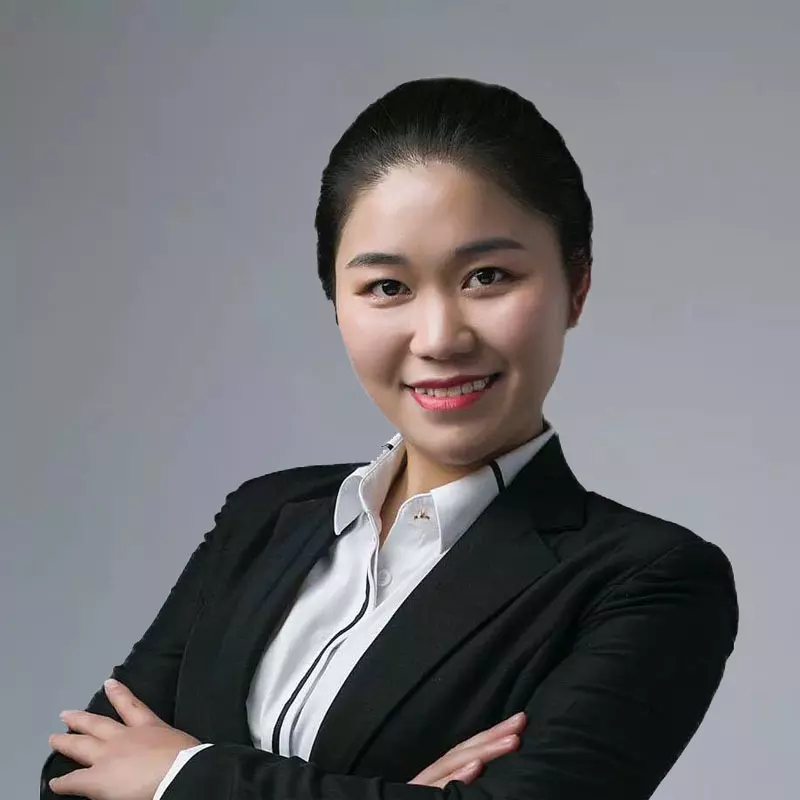Never Xiong